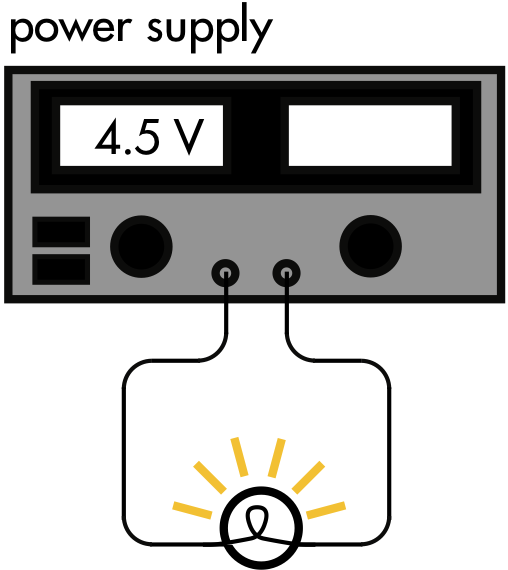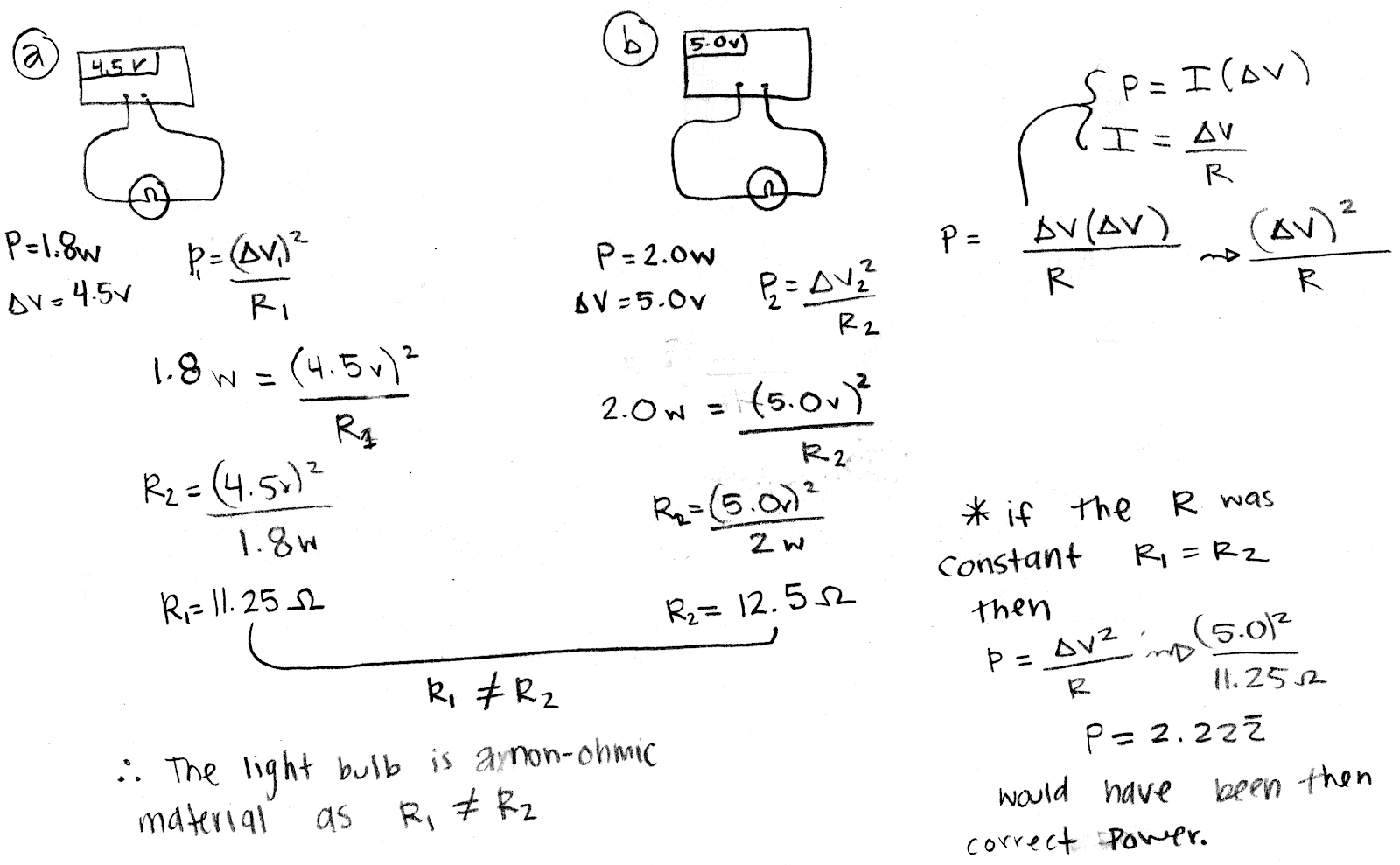## 20180505

### Physics midterm problem: non-ohmic light bulb

Physics 205B Midterm 2, spring semester 2018
Cuesta College, San Luis Obispo, CAA power supply with a built-in voltmeter is connected to a light bulb. When 4.5 V is applied to the light bulb, it uses 1.8 W of power. When the voltage applied is increased to 5.0 V, the light bulb then uses 2.0 W of power. Demonstrate how these measurements show that the light bulb is non-ohmic (that is, its resistance is not constant). Explain your reasoning using Kirchhoff's rules, Ohm's law, and properties of electrical power.

• p:
Correct. Demonstrates that the resistance of the light bulb is not constant by calculating the different values it has for each different applied voltage value.
• r:
Nearly correct, but includes minor math errors.
• t:
Nearly correct, but approach has conceptual errors, and/or major/compounded math errors.
• v:
Implementation of right ideas, but in an inconsistent, incomplete, or unorganized manner. Some attempt at applying Kirchhoff's rules, Ohm's law, and properties of electrical power.
• x:
Implementation of ideas, but credit given for effort rather than merit. No clear attempt at applying Kirchhoff's rules, Ohm's law, and properties of electrical power.
• y:
Irrelevant discussion/effectively blank.
• z:
Blank.
Sections 30882, 30883
Exam code: midterm02iFtW
p: 28 students
r: 1 student
t: 1 student
v: 4 students
x: 0 student
y: 0 students
z: 0 students

A sample "p" response (from student 3693):